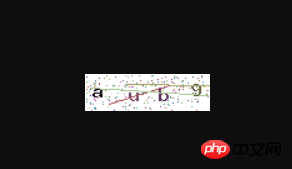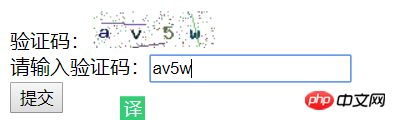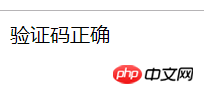1）一个长方形的底图

2）验证码的内容（数字，数字+英文，中文）

3）各色的干扰点

4）长短颜色不一的干扰线段

1.长方形的底图

我们所见的验证码一般都是一个100*30px的底图，有的中文验证码底图会大一点（200*60px）,而这个底图是如何来的呢？

在php语言中，有关于图像的处理有专门的函数库--GD库，不了解的先查询手册---php-图像处理和 GD

了解该库后，我们还要确保自己的php中带有该库且该库开启。一般php都会带有且默认开启。我们可以用phpinfo();函数查看是否有该库存在，如果GD库已有但未开启则需要在php.ini extension=php_gd2.dll前面的分号去掉，重启apache。

制作验证码所需的该GD库函数如下：

```imagecreatetruecolor(int \$width , int \$height) — 新建一个真彩色图像(网页黑色背景);

imagecolorallocate( resource \$image , int \$red , int \$green , int \$blue ) — 为一幅图像分配颜色(RGB);

imagefill(resource \$image , int \$x , int \$y , int \$color ) — 区域填充(在\$image上从坐标\$x,\$y处开始填充颜色\$color)；

imagestring(resource \$image , int \$font , int \$x , int \$y , string \$s , int\$col) — 水平地画一行字符串（ 用 \$col 颜色将字符串 \$s 画到 \$image 所代表的图像的\$x，\$y 坐标处）；

imagettftext( resource \$image , float \$size , float \$angle , int \$x , int\$y , int \$color , string \$fontfile , string \$text)— 用 TrueType 字体向图像写入文本(\$angle表示字符偏转的角度)

imagesetpixel(resource \$image , int \$x , int \$y , int \$color) — 画一个单一像素点（在 \$image 图像中用 \$color 颜色在\$x，\$y 坐标上画一个点）,即验证码上的干扰点；

imageline(resource \$image , int \$x1 , int \$y1 , int \$x2 , int \$y2 , int\$color) — 画一条线段(两点确定一条直线，用于验证码干扰线段)；

imagepng(resource \$image , string \$filename) — 以 PNG 格式将图像输出到浏览器或文件(将 GD 图像流（\$image）以 PNG 格式输出到标准输出（通常为浏览器），或者如果用\$filename 给出了文件名则将其输出到该文件。)

imagedestroy(resource \$image) — 销毁一图像(资源销毁)```

上面这些函数，足够完成我们的验证码，我们先完成验证码底图的制作：

```<?php
session_start();//开启session。session功能保存验证码内容用于验证输入验证码是否正确

\$image = imagecreatetruecolor(100,30);//生成一个100*30px的真彩色图像
\$bgcolor = imagecolorallocate(\$image,255,255,255);//白色
imagefill(\$image,0,0,\$bgcolor);//将\$image完全填充为白色，即白色底图```

2.验证码的内容（数字，数字+英文，中文）

100*30底图完成后，我们应在底图上添加验证码内容：

a.数字验证码：

```\$captch_code = '';//保存验证码字符串，后面存入session用于session验证
//验证码随机四个数字
for (\$i=0;\$i<4;\$i++){
\$fontsize = 6;//使用字体
\$fontcolor = imagecolorallocate(\$image,rand(0,120),rand(0,120),rand(0,120));//数字颜色，因为底图为白色，所以四个数字随机区域为深色区域
\$fontcontent = rand(0,9);//0-9随机数字
\$captch_code .= \$fontcontent;//字符串拼接到\$captch_code

\$x=(\$i*100/4) + rand(5,10);//数字在底图上的x坐标，利用变量\$i使数字保持间距
\$y=rand(5,10);//y坐标

imagestring(\$image,\$fontsize,\$x,\$y,\$fontcontent,\$fontcolor);//将数字\$fontcontent以颜色\$fontcolor、字体大小\$fontsize画到\$image的\$x,\$y处
}
\$_SESSION['authcode'] = \$captch_code;//保存验证信息，等待校验```

b.数字+字母验证码：

```\$captch_code = '';//保存验证码字符串，后面存入session用于session验证
//数字字母混合
for (\$i=0;\$i<4;\$i++){
\$fontsize = 6;
\$fontcolor = imagecolorallocate(\$image,rand(0,120),rand(0,120),rand(0,120));
\$data = 'qwertyuiplkjhgfdsazxcvbnm123456789';//所有数字字母组成的字符串，为用户考虑去除难辨识的o和0；
\$fontcontent = substr(\$data,rand(0,strlen(\$data)),1);//从\$data中随机取出一个字符
\$captch_code .= \$fontcontent;//字符串拼接到\$captch_code

\$x=(\$i*100/4) + rand(5,10);
\$y=rand(5,10);

imagestring(\$image,\$fontsize,\$x,\$y,\$fontcontent,\$fontcolor);
}

\$_SESSION['authcode'] = \$captch_code;//保存验证信息```

c.中文验证码：

注意，中文验证码必须导入支持中文的字体文件，windows系统可以在控制面板-字体中查找。

```\$captch_code = '';//保存验证码，用于session验证

\$fontface = 'simkai.ttf';//字体文件
//汉字库，就是可能随机出现在底图上的所有中文内容
\$str = "肥哦富农卫生费呢哦肥婆飞马珀耳风光迷人惠恩或嗯哦分配热攻击内容个人哦法二夫人很愉快热望特如果以后突然语句一进门就十足的傻瓜他会放过冰女冰女并承诺纷纷热狗后台交互同意木有就如同过河去啊封闭设备覅为法国梧桐耶夫北京市的发表都被你为防备分别是的小蜜蜂内裤我放不下的尽快发呢我尽快发布弄撒的烦恼腹部欸数量";
\$strdb = str_split(\$str,3); //把字符串切割成数组，一个汉字占用三个字节。

//四个中文验证码
for (\$i=0;\$i<4;\$i++){
\$fontcolor = imagecolorallocate(\$image,rand(0,120),rand(0,120),rand(0,120));

\$index = rand(0,count(\$strdb)-1);//产生随机数，\$strdb下标是从0开始的，所以count()的值-1.
\$cn = \$strdb[\$index];//随机选取汉字
\$captch_code .= \$cn;//汉字拼接到\$captch_code，用于验证

//imagestring()函数不支持中文，此处改用imagettftext();
imagettftext(\$image,mt_rand(20,24),mt_rand(-60,60),(40*\$i+20),mt_rand(30,35),\$fontcolor,\$fontface,\$cn);
}
\$_SESSION['authcode'] = \$captch_code;//保存验证信息```

3.各色的干扰点

```//增加干扰元素(点)，干扰点不可太少也不应太多，200适中
for (\$i=0;\$i<200;\$i++){
\$pointcolor = imagecolorallocate(\$image,rand(50,200),rand(50,200),rand(50,200));//干扰点的颜色不应该干扰用户正常阅读验证码内容，所以随机浅色区域
imagesetpixel(\$image,rand(1,99),rand(1,29),\$pointcolor);
}```

4.长短颜色不一的干扰线段

```//干扰元素(线)
for (\$i=0;\$i<3;\$i++){
\$linecolor = imagecolorallocate(\$image,rand(80,200),rand(80,200),rand(80,200));
imageline(\$image,rand(1,99),rand(1,29),rand(1,99),rand(1,29),\$linecolor);
}```

```header('content-type:image/png');//输出前添加文件头信息
imagepng(\$image);

//end 资源销毁
imagedestroy(\$image);``````<form action="captcha_check.php" method="post">
<!--    验证码路径添加一个随机数参数，防止路径图片被缓存-->
验证码：<img src="captcha.php?r=<?php echo rand() ?>"><br>
请输入验证码：<input type="text" name="captcha"><br>
<input type="submit" value="提交">
</form>```

```<?php
session_start();

//\$_REQUEST接收用户输入的验证码
if (\$_REQUEST['captcha'] == \$_SESSION['authcode']){
echo '验证码正确';
}else{
echo '验证码错误';
}```